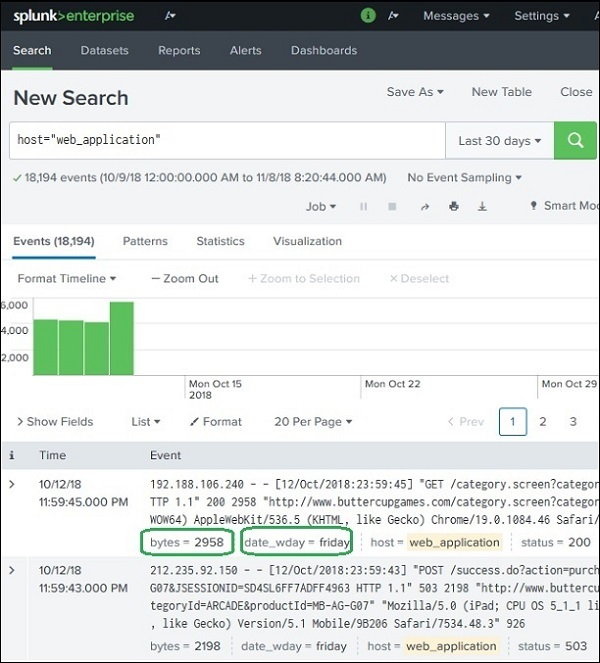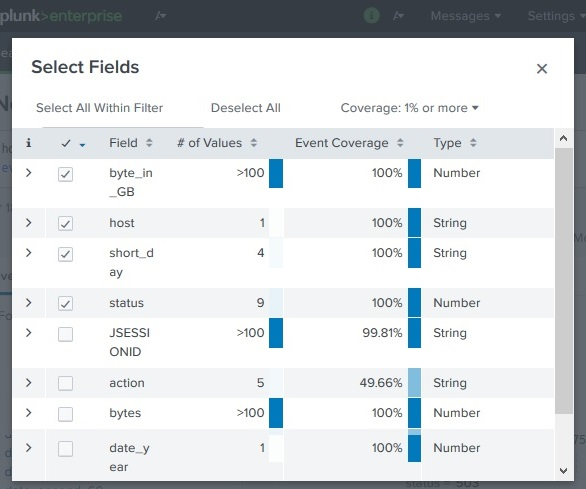# Splunk - Calculated Fields

Many times, we will need to make some calculations on the fields that are already available in the Splunk events. We also want to store the result of these calculations as a new field to be referred later by various searches. This is made possible by using the concept of calculated fields in Splunk search.

A simplest example is to show the first three characters of a week day instead of the complete day name. We need to apply certain Splunk function to achieve this manipulation of the field and store the new result under a new field name.

## Example

The Web_application log file has two fields named bytes and date_wday. The value in the bytes field is the number of bytes. We want to display this value as GB. This will require the field to be divided by 1024 to get the GB value. We need to apply this calculation to the bytes field.

Similarly, the date_wday displays complete name of the week day. But we need to display only the first three characters.

The existing values in these two fields is shown in the image below −## Using the eval Function

To create calculated field, we use the eval function. This function stores the result of the calculation in a new field. We are going to apply the below two calculations −

```# divide the bytes with 1024 and store it as a field named byte_in_GB
Eval byte_in_GB = (bytes/1024)

# Extract the first 3 characters of the name of the day.
Eval short_day = substr(date_wday,1,3)
```

We add new fields created above to the list of fields we display as part of the search result. To do this, we choose All fields options and tick check mark against the name of these new fields as shown in below image −## Displaying the calculated Fields

After choosing the fields above, we are able to see the calculated fields in the search result as shown below. The search query displays the calculated fields as shown below −